In mathematics, a self-adjoint operator is a densely defined linear operator mapping a complex Hilbert space onto itself and which is invariant under the unary operation of taking the adjoint. That is, if A is an operator with a domain$H_0$ which is a dense subspace of a complex Hilbert space H then it is self-adjoint if$A=A^*$, where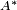$A^*$ denotes the adjoint operator of A. Note that the adjoint of any densely defined linear operator is always well-defined (in fact, the denseness of the domain of an operator is necessary for the existence of its adjoint) and two operators A and B are said to be equal if they have a common domain and their values coincide on that domain.

On an infinite dimensional Hilbert space, a self-adjoint operator can be thought of as the analogy of a real symmetric matrix (i.e., a matrix which is its own transpose) or a Hermitian matrix in (i.e., a matrix which is its own Hermitian transpose) when these matrices are viewed as (bounded) linear operators on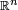$\mathbb{R}^n$ and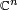$\mathbb{C}^n$, respectively.

## Special properties of a self-adjoint operator

The self-adjointness of an operator entails that it has some special properties. Some of these properties include:

1. The eigenvalues of a self-adjoint operator are real. As a special well-known case, all eigenvalues of a real symmetric matrix and a complex Hermitian matrix are real.

2. By the von Neumann’s spectral theorem, any self-adjoint operator X (not necessarily bounded) can be represented as$X=\int_{-\infty}^{\infty} x E^X(dx),$

where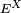$E^X$ is the associated spectral measure of X (in particular, a spectral measure is a Hilbert space projection operator-valued measure).

3. By Stone’s Theorem, for any self-adjoint operator X the one parameter unitary group$U=\{U_t\}_{t \in \mathbb{R}}$ defined by$U_t=\int_{-\infty}^{\infty} e^{-itx}\, E^X(dx)$, where$E^X$ is the spectral measure of X, satisfies:$\frac{dU_t}{dt} u=-iXU_t u =U_t(-iX)u,$

for all u in the domain of X. One says that the operator -iX is the generator of the group U and writes:$U_t=e^{-itX},\,\,t \in \mathbb{R}$.

As mentioned above, a simple instance of a self-adjoint operator is a Hermitian matrix.

For a more advanced example consider the complex Hilbert space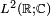$L^2(\mathbb{R};\mathbb{C})$ of all complex-valued square integrable functions on$\mathbb{R}$ with the complex inner product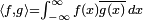$\langle f,g\rangle=\int_{-\infty}^{\infty}f(x)\overline{g(x)}\,dx$, and the dense subspace$C^{\infty}_0(\mathbb{R};\mathbb{C})$ of$L^2(\mathbb{R};\mathbb{C})$ of all infinitely differentiable complex-valued functions with compact support on$\mathbb{R}$. Define the operators Q, P on$C^{\infty}_0(\mathbb{R};\mathbb{C})$ as: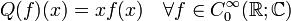$Q(f)(x)= xf(x) \quad \forall f \in C^{\infty}_0(\mathbb{R};\mathbb{C})$

and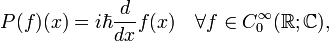$P(f)(x)=i \hbar \frac{d}{dx}f(x) \quad \forall f \in C^{\infty}_0(\mathbb{R};\mathbb{C}),$

where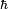$\hbar$ is the real valued Planck's constant. Then Q and P are self-adjoint operators satisfying the commutation relation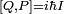$[Q,P]=i\hbar I$ on$C^{\infty}_0(\mathbb{R};\mathbb{C})$, where I denotes the identity operator. In quantum mechanics, the pair Q and P is known as the Schrödinger representation, on the Hilbert space$L^2(\mathbb{R};\mathbb{C})$, of canonical conjugate position and momentum operators q and p satisfying the canonical commutation relation (CCR)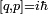$[q,p]=i\hbar$.Some content on this page may previously have appeared on Citizendium.